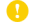Online ISSN : 1880-9014
Print ISSN : 0532-8799
ISSN-L : 0532-8799

ジャーナル フリー

2018 年 65 巻 7 号 p. 407-413J-STAGE : jjspm 66 1 pp.60-

Solid solution strengthening effect by oxygen (O) and nitrogen (N) atoms of α-titanium (Ti) materials was quantitatively evaluated using Labusch model by consideration of the experimental data. When using Labusch model to predict solid solution strengthening improvement, an application of the isotropic strains by solute elements is generally assumed to estimate Fm value, which is the maximum interaction force between the solute atoms and dislocations. It is, however, difficult to exactly calculate Fm value for α-Ti materials with O and N solute atoms because the anisotropic strains are induced in α-Ti crystal with hcp structure by these elements. In this study, Fm value was experimentally derived from the relationship between 0.2% yield stress and solute elements (O and N atoms) content of Ti sintered materials. As a result, the strengthening improvement was proportional to c2/3/Sf (c: soluted atom content, Sf: Schmid factor), and its factor of proportionality of Ti-O and Ti-N materials was 4.17 × 103 and 3.29 × 103, respectively. According to this analysis, it was clarified that Fm value of Ti-O and Ti-N materials was 6.22 × 10−10 and 5.21 × 10−10, respectively, and then the estimated strengthening improvement by using these values was significantly agreed with the experimental results of Ti sintered materials with O and N solution atoms.著者関連情報